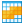You are viewing the RapidMiner Studio documentation for version 8.1 - Check here for latest version

#Grouped ANOVA (RapidMiner Studio Core)

## Synopsis

This operator performs an ANOVA significance test for the user-specified attribute (numerical) based on the groups defined by the user-specified attribute (nominal). ANOVA is a general technique that can be used to test the hypothesis that the means among two or more groups are equal, under the assumption that the sampled populations are normally distributed.

## Description

The Grouped ANOVA operator creates groups of the input ExampleSet based on the grouping attribute which is specified by the group by attribute parameter. For each of the groups the mean and variance of the anova attribute is calculated and an ANalysis Of VAriance (ANOVA) is performed. The anova attribute is specified by the anova attribute parameter. It is important to note that the grouping attribute should be nominal and the anova attribute should be numerical. The result of this operator is a significance test result for the specified significance level (specified by the significance level parameter) indicating if the values for the attribute are significantly different between the groups defined by the grouping attribute.

ANalysis Of VAriance (ANOVA) is a statistical model in which the observed variance in a particular variable is partitioned into components attributable to different sources of variation. In its simplest form, ANOVA provides a statistical test of whether or not the means of several groups are all equal, and therefore generalizes a t-test to more than two groups. Doing multiple two-sample t-tests would result in an increased chance of committing a Type I error. For this reason, ANOVA is useful in comparing two, three, or more means. 'False positive' or a Type I error is defined as the probability that a decision to reject the null hypothesis will be made when it is in fact true and should not have been rejected. In the typical application of ANOVA, the null hypothesis is that all groups are simply random samples of the same population. This implies that all treatments have the same effect (perhaps none). Rejecting the null hypothesis implies that different treatments result in altered effects.

## Differentiation

###ANOVA Matrix

The ANOVA Matrix operator performs ANOVA significance test for all numerical attributes based on the groups defined by all the nominal attributes.

## Input

•example set (Data Table)

This input port expects an ExampleSet. It is the output of the Retrieve operator in the attached Example Process. The output of other operators can also be used as input. The ExampleSet should have both nominal and numerical attributes because this operator performs an ANOVA significance test for a specified numerical attribute based on the groups defined by a specified nominal attribute.

## Output

•significance (ANOVA Significance)

The ANOVA test is performed and the ANOVA significance test result is returned from this port.

•example set (Data Table)

The ExampleSet that was given as input is passed without changing to the output through this port. This is usually used to reuse the same ExampleSet in further operators or to view the ExampleSet in the Results Workspace.

## Parameters

• anova_attributeThe ANOVA is calculated for the attribute specified by this parameter based on the groups defined by the group by attribute parameter. It is compulsory that this attribute should be numerical. Range: string
• group_by_attributeGrouping is performed by the values of the attribute specified by this parameter. It is compulsory that this attribute should be nominal. Range: string
• significance_levelThis parameter specifies the significance level for the ANOVA calculation. Range: real
• only_distinctThis parameter indicates if only rows with distinct values of the aggregation attribute should be used for the calculation of the aggregation function. Range: boolean

## Tutorial Processes

### Grouped ANOVA of the Golf data set

The 'Golf' data set is loaded using the Retrieve operator. A breakpoint is inserted here so that you can view the ExampleSet. You can see that the ExampleSet has both nominal and numerical attributes. The Grouped ANOVA operator is applied on this ExampleSet. The anova attribute and group by attribute parameter are set to 'Humidity' and 'Play' respectively. This operator performs an ANOVA significance test for the 'Humidity' attribute based on the groups defined by the 'Play' attribute. The result of the ANOVA significance test can be viewed in the Results Workspace.